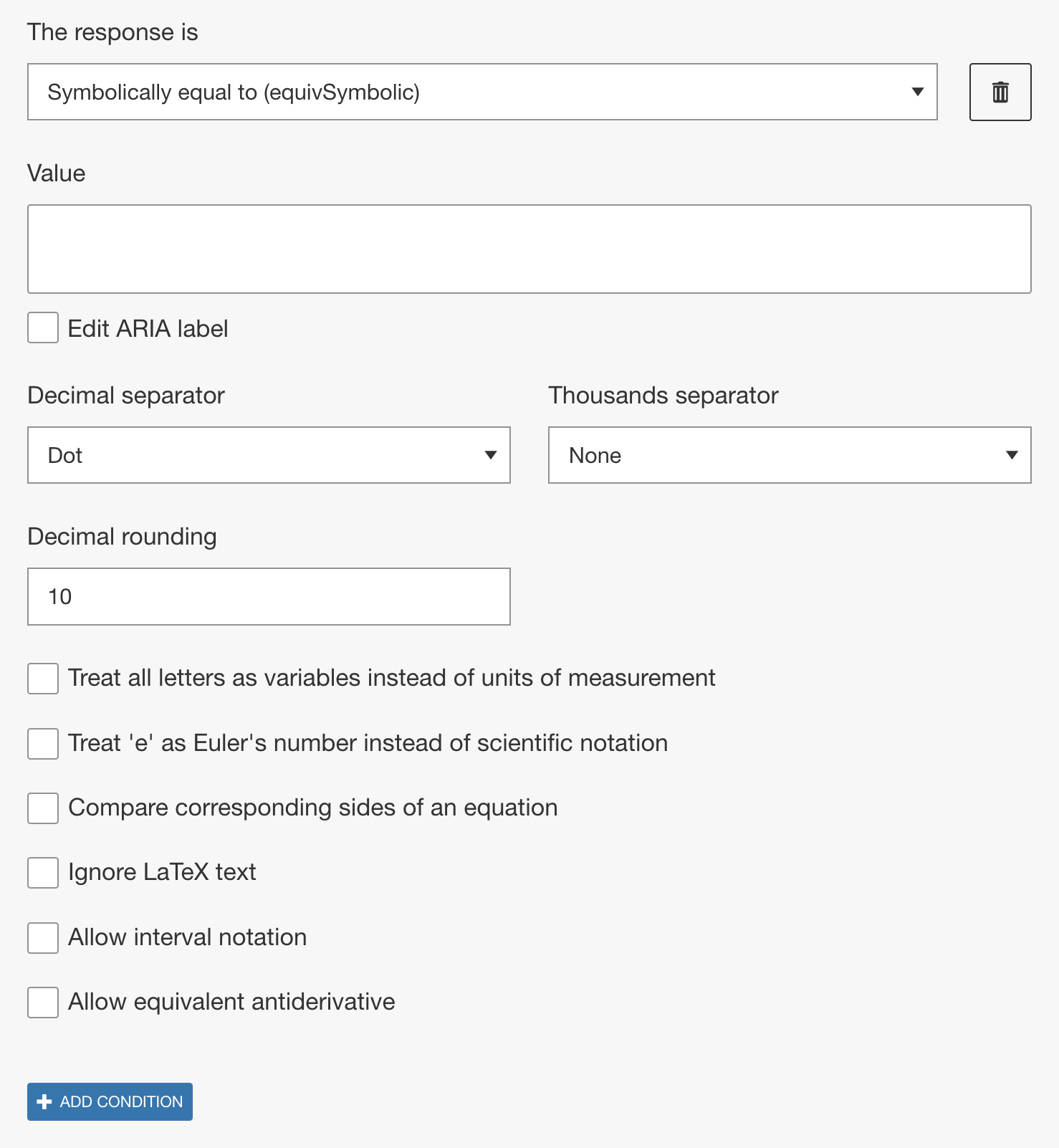# Symbolically equal to (equivSymbolic)

## Overview

The Symbolically equal to (equivSymbolic) method checks whether the value entered by the learner is mathematically equivalent to the sample answer the Author has set in the value field regardless of the form. This method is very flexible and is capable of performing arithmetical, algebraic, exponential, logarithmic, and trigonometric manipulations to recognize the equivalence of values expressed in different forms.

Use equivSymbolic when you do not require the learner to express their answer in a specific format. The method equivSymbolic accepts a wide range of inputs including decimals, fractions, variables, percentages, trigonometric, logarithmic, exponential, vector and matrix expressions.Figure 1: Setting correct answer for equivSymbolic in Math Question type.

• Decimal and thousands separator
Authors can specify what separators should be recognized by the engine and can be used by the learner. From the Decimal separator drop-down either a dot or a comma can be selected. Depending on the selection of the decimal separator, from the Thousand Separator drop-down dot, comma, and/or space can be selected.
• Decimal rounding
This option specifies the number of decimal places the learner response and the Author value are rounded to before comparison. The maximum and default value is 10.
• Treat all letters as variables instead of units of measurement
When enabled the engine will treat all letters as variables. This is useful in cases where you want to use letters as variables that the math engine may normally recognize as units of measurement. See article on the list of supported units
• Treat 'e' as Euler's number instead of scientific notation
This option treats the variable 'e' as Euler's number. This overrides the default interpretation of 'e' as scientific notation.
• Compare corresponding sides of an equation
With this option enabled, when comparing two equations the engine will compare the corresponding sides of each equation individually rather than the whole equations. For example, if the authored value was `y=x+1` and the option was selected, `y-x=1` would no longer be a correct learner response.
• Ignore LaTex text
This option refers to LaTeX text only and when enabled the engine will ignore any LaTeX text in the authored value and learner response when comparing for equivalency. For example, any text added in a Text block by the author or units entered by the keypad are set as LaTex text and would be ignored.
• Allow interval notation
This option must be enabled when the learner is expected to insert interval notation in the response area, ie `[1, 4)`. Without this option enabled, the response may not validate correctly especially when mis-matched brackets are expected to be used.
• Allow equivalent antiderivative
This options allows learner response to differ from the authored value by a constant. Use this option to score answers to indefinite integrals in Calculus courses. Response values must include a lower or uppercase C to designate a constant of integration. Additionally authors must specify the integration variable. The default value is set as x and the engine only accepts single letters.